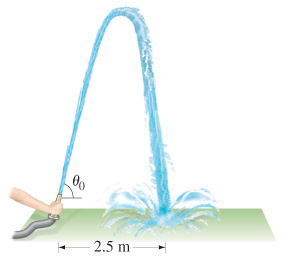# Problem: A fire hose held near the ground shoots water at a speed of 6.5 m/s.(A) At what angle(s) should the nozzle point in order that the water land 2.5 m away?(B) Why are there two different angles?(C) Sketch the trajectory for the 18 angle. Plot points starting at x = 0 m in 0.5 m increments and at the highest point of the trajectory.(D) Sketch the trajectory for the 72 angle. Plot points starting at x = 0 m in 0.5 m increments and at the highest point of the trajectory.

###### FREE Expert Solution

In this problem, we are projecting an object (water) upward at an angle. The object falls down after reaching the maximum height and hits the ground. We are given the range covered and asked to solve for the angle. We know that the angle and range are related by equations derived in symmetrical launch. Thus, this is a symmetrical launch problem.

89% (474 ratings)###### Problem Details

A fire hose held near the ground shoots water at a speed of 6.5 m/s.

(A) At what angle(s) should the nozzle point in order that the water land 2.5 m away?

(B) Why are there two different angles?

(C) Sketch the trajectory for the 18 angle. Plot points starting at x = 0 m in 0.5 m increments and at the highest point of the trajectory.

(D) Sketch the trajectory for the 72 angle. Plot points starting at x = 0 m in 0.5 m increments and at the highest point of the trajectory.

Frequently Asked Questions

What scientific concept do you need to know in order to solve this problem?

Our tutors have indicated that to solve this problem you will need to apply the Symmetrical Launch concept. You can view video lessons to learn Symmetrical Launch. Or if you need more Symmetrical Launch practice, you can also practice Symmetrical Launch practice problems.

What professor is this problem relevant for?

Based on our data, we think this problem is relevant for Professor Pascuzzi's class at SUNY.